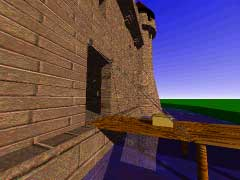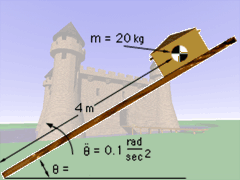Ch 2. Particle Force and Acceleration Multimedia Engineering Dynamics Rect.Coord. Normal/Tang. Coord. PolarCoord. Orbital Mechanics Computational Mechanics
 Chapter - Particle - 1. General Motion 2. Force & Accel. 3. Energy 4. Momentum - Rigid Body - 5. General Motion 6. Force & Accel. 7. Energy 8. Momentum 9. 3-D Motion 10. Vibrations Appendix Basic Math Units Basic Equations Sections Search eBooks Dynamics Fluids Math Mechanics Statics Thermodynamics Author(s): Kurt Gramoll ©Kurt GramollDYNAMICS - CASE STUDY IntroductionProblem Animation The court jester forgot to remove the King's treasure chest from the drawbridge. The jester is now closing the drawbridge, and if the chest falls and breaks, it will cost him his head! What is known: The mass of the chest is 20 kg. Initially, the chest is located 4 m from the foot of the drawbridge. The drawbridge starts from rest in a horizontal position and rotates with a constant angular acceleration of 0.1 rad/s2. QuestionProblem Parameters If the drawbridge reaches an angle of 30° before the jester can stop it, what is the coefficient of static friction that must exist between the chest and the drawbridge so that the chest will not slide down the drawbridge. Approach Using the expressions for acceleration in polar coordinates (derived in Curvilinear Motion: Polar Coordinates section), express Newton's Second Law in terms of polar coordinates. Determine the normal force exerted on the chest by the bridge at 30°. Use the normal force at 30° to find the minimum static coefficient of friction that must exist.

Practice Homework and Test problems now available in the 'Eng Dynamics' mobile app
Includes over 400 problems with complete detailed solutions.
Available now at the Google Play Store and Apple App Store.